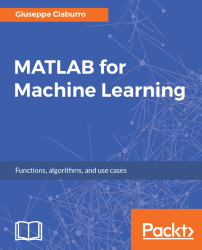•#### MATLAB for Machine Learning#### Overview of this book

MATLAB is the language of choice for many researchers and mathematics experts for machine learning. This book will help you build a foundation in machine learning using MATLAB for beginners. You’ll start by getting your system ready with t he MATLAB environment for machine learning and you’ll see how to easily interact with the Matlab workspace. We’ll then move on to data cleansing, mining and analyzing various data types in machine learning and you’ll see how to display data values on a plot. Next, you’ll get to know about the different types of regression techniques and how to apply them to your data using the MATLAB functions. You’ll understand the basic concepts of neural networks and perform data fitting, pattern recognition, and clustering analysis. Finally, you’ll explore feature selection and extraction techniques for dimensionality reduction for performance improvement. At the end of the book, you will learn to put it all together into real-world cases covering major machine learning algorithms and be comfortable in performing machine learning with MATLAB.
Title PageCreditsForewordwww.PacktPub.comCustomer FeedbackPrefaceFree Chapter
Getting Started with MATLAB Machine LearningImporting and Organizing Data in MATLABFrom Data to Knowledge DiscoveryFinding Relationships between Variables - Regression TechniquesPattern Recognition through Classification AlgorithmsIdentifying Groups of Data Using Clustering MethodsSimulation of Human Thinking - Artificial Neural NetworksImproving the Performance of the Machine Learning Model - Dimensionality ReductionMachine Learning in Practice## Summary

This final chapter has served us to revise the concepts learned in previous chapters; this time, without any introductions, but starting with a real-life case and analyzing the workflow that allows us to extract knowledge from a database.

In this chapter, we started with solving a fitting problem. We created a model that allows us to calculate the concrete compressive strength according to the ingredients used in the mixture. We learned how to import data in the MATLAB workspace and how to prepare it for subsequent analysis. Then, we resolved a fitting problem using Neural Network Toolbox.

Then, we learned how to use neural network to classify pattern. In this study, we created a model that allows us to classify thyroid diseases according to a lot of patient data. This time, we used a dataset that was already available in the MATLAB distribution. We also learned to build and understand the confusion matrices and the ROC.

Finally, we performed a clustering analysis. The purpose of this...